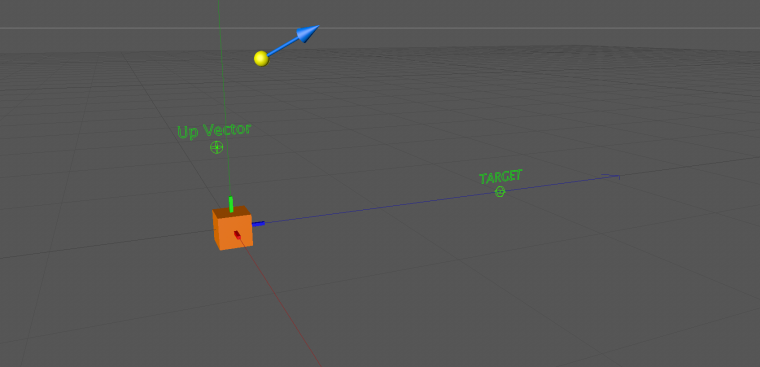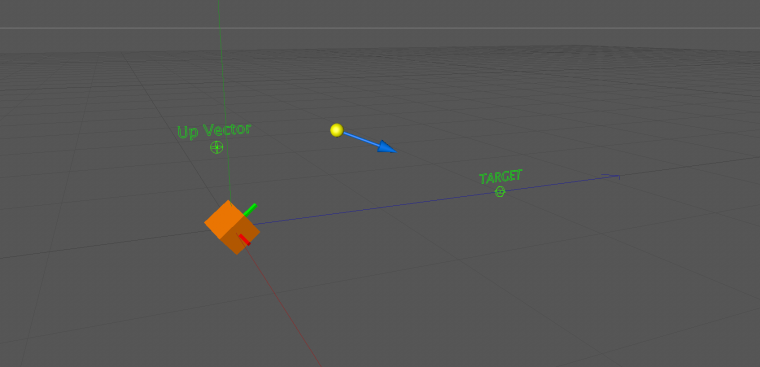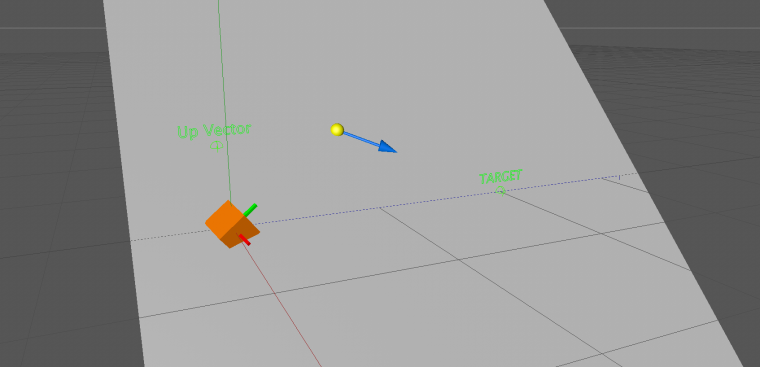# Aligning a ray origin and direction towards a target by rotating a parent matrix

• Not really sure if this is the right forum or not for this kind of thing... If it isn't, apologies in advance.

I'm trying to figure out how to align some objects in 3D space and I was wondering if anyone could help me out here. Generating lookat matrices is easy enough, but this appears to be something else entirely.

What I'm trying to do is to take one matrix as parented to another, and rotate the parent matrix so that a specific axis of the child matrix is pointing towards a target with an up vector to align the entire thing. The catch is that the child matrix can move around (and rotate), plus the target point and up vectors are going to change as well- which is why I'm trying to cram this into a plugin instead (albeit a rather specialized plugin).

Here's some example images of exactly what I'm trying to achieve:

In this image, the yellow sphere and blue arrow are what I need to align towards the target by rotating the matrix of the orange cube.Here they are aligned (which shows how the orange cube has been rotated until the blue arrow is pointing directly at the target):And here's an image with a plane slicing through the orange cube's origin, the up vector, the yellow sphere, and the target (which demonstrates how I need to align the entire system):I'm guessing this is just a matter of performing a whole bunch of space transforms and some dot/cross vector math, but I'll be damned if I can even figure out where to start. Originally I thought this might be solvable using simple trig (since the angle between the blue arrow and direction towards the orange cube will always be static, and since you know the distance from the yellow sphere to the orange cube and from the orange cube to the target point, you can derive the rest of the angles of the triangle that forms)- but that doesn't solve the fact that the orange cube needs to be rotated in such a way that the blue arrow always lands up pointing at the target.

How should I go about solving this problem?

Cheers,
-CMPX

• Hi,

it's perfectly ok to discuss such "exercises" in this forum. I just moved it into the General Programming category, as it does not seem C4D specific.
We need to leave this question to our community. Maybe after DevKitchen one of us is curious enough to look into it in his spare time, no promises made though.

I added a tag to your thread, see Read Before Posting. Also turned this thread into a question, see Q&A New Functionality.

Cheers,
Andreas

• Hi @cmpxchg8b and @a_block ,
I don't know much about matrix, but here is what I found.
If the `point with arrow` should rotate to aim, then all the possible position of the point must lay on the `sphere surface` which sphere's center is the parent object.
And one point and a vector can form a line in space.
So the question becomes a line-sphere intersection problem.
After solving the equations, we can get 2 points: `original point` and `transformed point(s)`.
After that, we can find the transform matrix between 2 points.